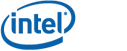# Coordinate Concept

The coordinate concept tag is coordinate_concept

To register a user defined type as a model of coordinate concept, specialize the geometry concept meta-function for that type.  In the example below CCoordinate is registered as a model of coordinate concept.

template <>
struct geometry_concept<CCoordinate> { typedef coordinate_concept type; };

The coordinate type is expected to be integral and built-in numerical data types such as float and int already have concept type traits specializations in the library.  In the coordinate traits are type definitions for related types are provided to allow the library to choose the best type to cast to under various circumstances.  The definition of coordinate_traits and its specialization for int are shown below.

template <typename T>
struct coordinate_traits {};

template <>
struct coordinate_traits<int> {
typedef int coordinate_type;
typedef long double area_type;
typedef long long manhattan_area_type;
typedef unsigned long long unsigned_area_type;
typedef long long coordinate_difference;
typedef long double coordinate_distance;
};

By making use of the coordinate traits of int the library is able to avoid overflow and handle the normal issues encountered when programming integer geometry.  For the out of the ordinary issues there is a special meta-function that provides the library with a numerical type suitable for exact numerical calculations.  It defaults to the highest precision data type available in most compilers, long double, but can be overridden by specializing for a particular coordinate type.  Use of gmp multi-precision rational or similar data type is recommended for numerically robust calculations in the general polygon algorithms.

template <typename T>
struct high_precision_type {
typedef long double type;
};

There is only one generic function on coordinate concepts, Euclidean distance.

template <typename coordinate_type_1, typename coordinate_type_2>
coordinate_difference euclidean_distance(coordinate_type_1, coordinate_type_2)

This function returns the absolution value of the difference between the two coordinates.

Note: older versions of the stl define a fully generic distance(T, T) function for computing the difference between two iterators.  We were forced to name our distance function euclidean_distance to avoid name collision.

The Algorithmic C ac_int<128> is an example of a user defined coordinate data type that satisfies the coordinate concept.  In general a data type should define std::numeric_limits and be integer-like.  Floating point coordinate types are not supported by all the algorithms and generally not suitable for use with the library at present.

Copyright: Copyright © Intel Corporation 2008-2010. Distributed under the Boost Software License, Version 1.0. (See accompanying file LICENSE_1_0.txt or copy at http://www.boost.org/LICENSE_1_0.txt)